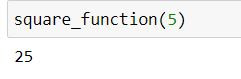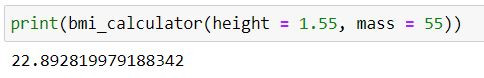Search
•Ekta Aggarwal

# User-Defined functions (UDFs) in Python

When you need to do some computations multiple times, instead of writing the same code N number of times, a good practise is to write the code chunk once as a function and then call the function with a single line of code.

In this tutorial, we shall be covering all the aspects of creating a user defined function (UDF) in Python.

A UDF can be used to:

• print something

• return values like scalar, lists, data frames,tuples etc.

• Do some mathematical computations on already existing dataset.

### Syntax:

```def function_name(parameter_list):
"""docstring""" <optional>
<Do something>
return( )   ------- Providing a return statement is optional```

def keyword tells Python that a UDF is being created.

parameter list: providing a parameter list is optional. We can pass multiple arguments in our parameter list separated by a comma (,)

docstring : The first text enclosed in triple quotes """ """ are called docstrings. Python does not evaluate them but it provides the context to the reader about what is the motive and utility of that function.

### Thing to remember:

Python is quite conservative about the indentation. Thus to indicate which lines form the UDF block you need to be very much careful with the spaces.

### Understanding with examples!

Example 1: Let us create our first function which prints "How are you doing today?"

Note that we are not defining any parameter_list in our print_function. Our function does not return anything!

```def print_function():
print("How are you doing today?")```

We have successfully created our function but how to see its output?

To see the output of a function we need to call it:

`print_function()`Example 2: Let us create our square_function which returns the square of a number.

In our function we are passing a parameter x, and we are assigning a local variable y which takes the value square of x. Finally our function is returning y.

```def square_function(x):
y = x**2;
return(y);

square_function(5)```

To get the square of 5, we call our square function as square_function(5)We can also store the output of our function in a new variable.

In the following code we are storing the output of square_function in the variable my_value.

```my_value = square_function(5);
print(my_value)```Example 3: Following function prints as well as returns the output!

```def square_function(x):
y = x**2;
#Prints the output
print("Square of my_num is:"+str(y))
#Returns the output
return(y);

square_function(5)```### Functions taking multiple parameters:

Example 4: Calculating BMI

BMI is calculated with the following formula:

BMI = mass/ (height * height)

Following function takes 2 parameters: mass and weight and then calculates the BMI.

```def bmi_calculator(mass,height):
bmi = mass/(height**2);
return(bmi);

bmi_calculator(70,1.88)```### Positional arguments

Note: The order of the parameters is important!

Since parameter list has mass as the first parameter and height as second, thus while calling the function you need to supply your parameter list in the same position.

`bmi_calculator(1.88,70) `

Above will lead to incorrect calculation as it will assign 1.88 to mass and 70 to height.

### Keyworded arguments

When your function has too many parameters then it becomes too difficult to remember the order of all the parameters thus while calling the functions you can specify the keyword (i.e. parameter name ) = value. These are called keyworded arguments.

```def bmi_calculator(mass,height):
bmi = mass/(height**2);
return(bmi)```

In the following code although we are specifying height followed by mass but our UDF will do correct calculations because we have specified our parameter name (keyword) with our values.

`print(bmi_calculator(height = 1.55, mass = 55))`### Default arguments

Like R, Python provides the utility to provide default values to the function parameters i.e. if the user does not specify the value then the default value can be used, but if the user specifies the value then the user-input value will be considered by the UDF.

Let us create our bmi_calculator where default height is 1.7

```def bmi_calculator(mass,height = 1.7):
bmi = mass/(height**2);
return(bmi);```

In the following code we are passing the value 70 to mass and 1.88 to height, in such a scenario default value of height will be over-written by 1.88

`print(bmi_calculator(70,1.88))`

In the case given below we are not passing any value for height, thus Python will consider height = 1.7

`print(bmi_calculator(70))`### Non default arguments must come before default arguments.

Let us define our function with default mass as 80.

```def bmi_calculator(mass = 80,height):
bmi = mass/(height**2);
return(bmi);```

Let us try calling our function by assigning 70 to mass and 1.88 to height.

`bmi_calculator(70,1.88)`

Python will throw up the SyntaxError: non-default argument follows default argument.

Thus for this we need to pass the value of height first and then mass.### Functions returning multiple values

A function can return a dataframe, list ,tuples and a scalar.

Let us create our function bmi_calculator which returns a tuple of 3 elements:

```def bmi_calculator(mass,height):
bmi = mass/(height**2);
my_tuple = (mass,height,bmi)
return(my_tuple);

print(bmi_calculator(70,1.88))```Since bmi_calculator returns 3 elements thus we can store each of these element as 3 new variables.

For example, we are creating 3 new variables my_mass, my_height and my_bmi in the following way:

```my_mass,my_height,my_bmi = bmi_calculator(70,1.88);
print(my_mass);
print(my_height);
print(round(my_bmi,2));```### Nested functions

A function inside a function is called a nested function:

In the following examples we are creating a function my_func which takes 3 input parameters and have defined a nested function square_func( ) inside it which returns the square of the number.

Note that indentation plays a big role here: For the second function we have specified another tab to dictate Python that it is a part of second function.

```def my_func(x1, x2, x3):
""" Indentation for first function"""
def square_func(x):
"""Indentation for second function"""
return x**2
return (square_func(x1), square_func(x2), square_func(x3))

print(my_func(1, 2, 3))```### args keyword

Now let us try to pass more arguments to the function:

`print(my_func(1, 2, 3,4, 5))`

Python has thrown the TypeError: which means that function can take only 3 arguments while you are passing 5.But it is not feasible that number of arguments are always known to a programmer in advance. Thus to mitigate this Python has args . Let us create the same function, where we are passing *args as our function parameter.

```def my_func(*args):
squared_tuple = [i**2 for i in args]
return tuple(squared_tuple)

my_func(1,2,3,4,5)```

*args parameter allows the programmer to pass non-keyworded arguments on which list operations can be carried out.### kwargs keyword

kwargs is the short form of keyword arguments. It is denoted by ** . It has a similar utility to args but it is not used for lists, rather it is used for working with dictionaries.

When number of items in our dictionary is not defined then we can use **kwargs.

Let us take an example to understand how a UDF with kwargs looks like:

```def my_func(**kwargs):
"""Printing Items in kwargs"""
print(kwargs.items())
"""Printing Keys in kwargs"""
print(kwargs.keys())
"""Printing Values in kwargs"""
print(kwargs.values())

for k,v in kwargs.items():
print(k," - ",v )```

Our above UDF will take a dictionary of varying number of keyworded arguments (i.e. items) and would print the items, keys, values and concatenate key and values.

Let us pass a dictionary with 2 items to our above UDF.

`my_func(country = "U.S.A", capital = "Washington D.C.")`To learn about args and kwargs in detail you can refer to this tutorial.

Tags:

See All